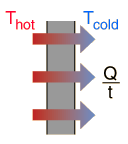# Heat Conduction

Heat transfer by conduction can be used to model heat loss through a wall. For a barrier of constant thickness, the rate of heat loss is given by:## Active formula

Enter data below and then click on the quantity you wish to calculate in the active formula above. Default values will be entered to avoid zero values for parameters, but all values may be changed. Note! The values will not be forced to be consistent until you click on something in the active formula to choose a calculation.Click on the quantity you want to calculate in the active formula above after entering data. For a wall of surface area A = m2 = ft2 and thickness d = cm = inches, thermal conductivity k = W/m°C = BTU/hr ft°F, Thot = °C = °F, Tcold = °C = °F, then the conduction heat loss rate is Q/t = watts = BTU/hr.
 Thermal conductivity table
 Discussion of thermal conductivity
Index

Heat transfer concepts

Heat transfer examples

 HyperPhysics***** Thermodynamics R Nave
Go Back